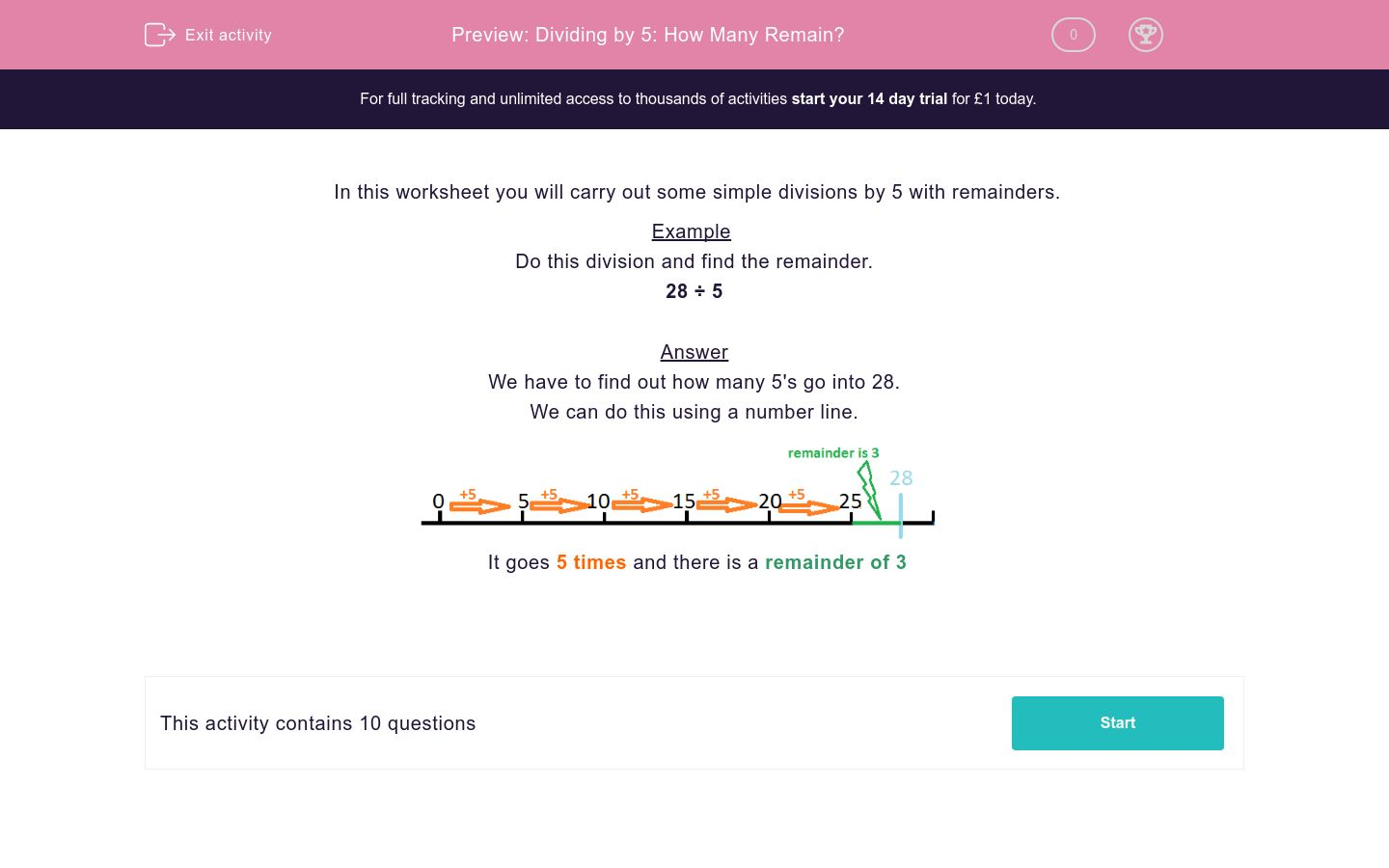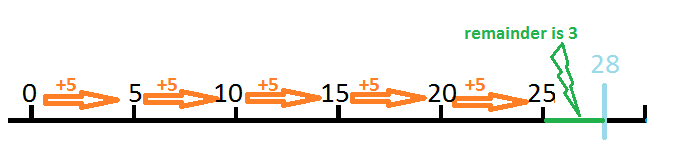# Dividing by 5: How Many Remain?

In this worksheet, students divide two-digit numbers by 5 and note down any remainders.Key stage:  KS 2

Curriculum topic:   Maths and Numerical Reasoning

Curriculum subtopic:   Mixed Problems

Difficulty level:### QUESTION 1 of 10

In this worksheet you will carry out some simple divisions by 5 with remainders.

Example

Do this division and find the remainder.

28 ÷ 5

We have to find out how many 5's go into 28.

We can do this using a number line.It goes 5 times and there is a remainder of 3

---- OR ----